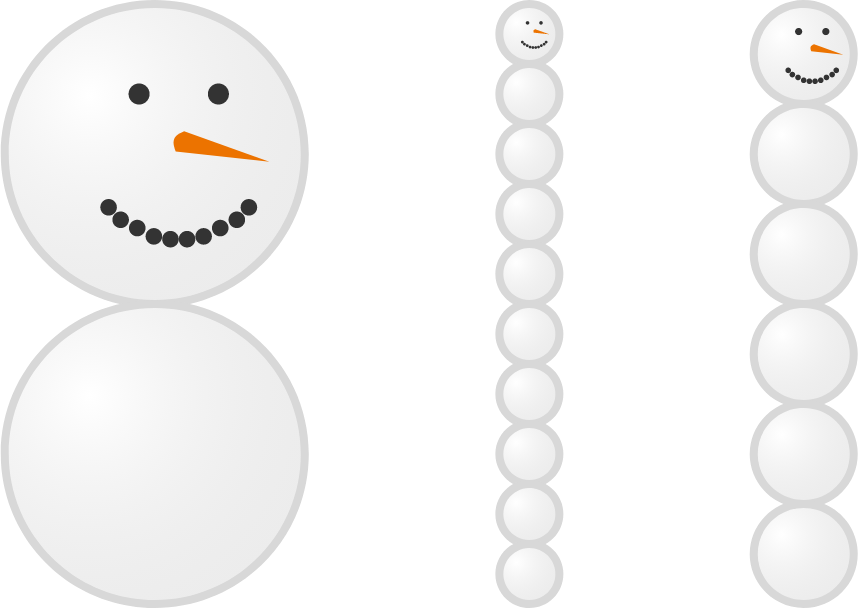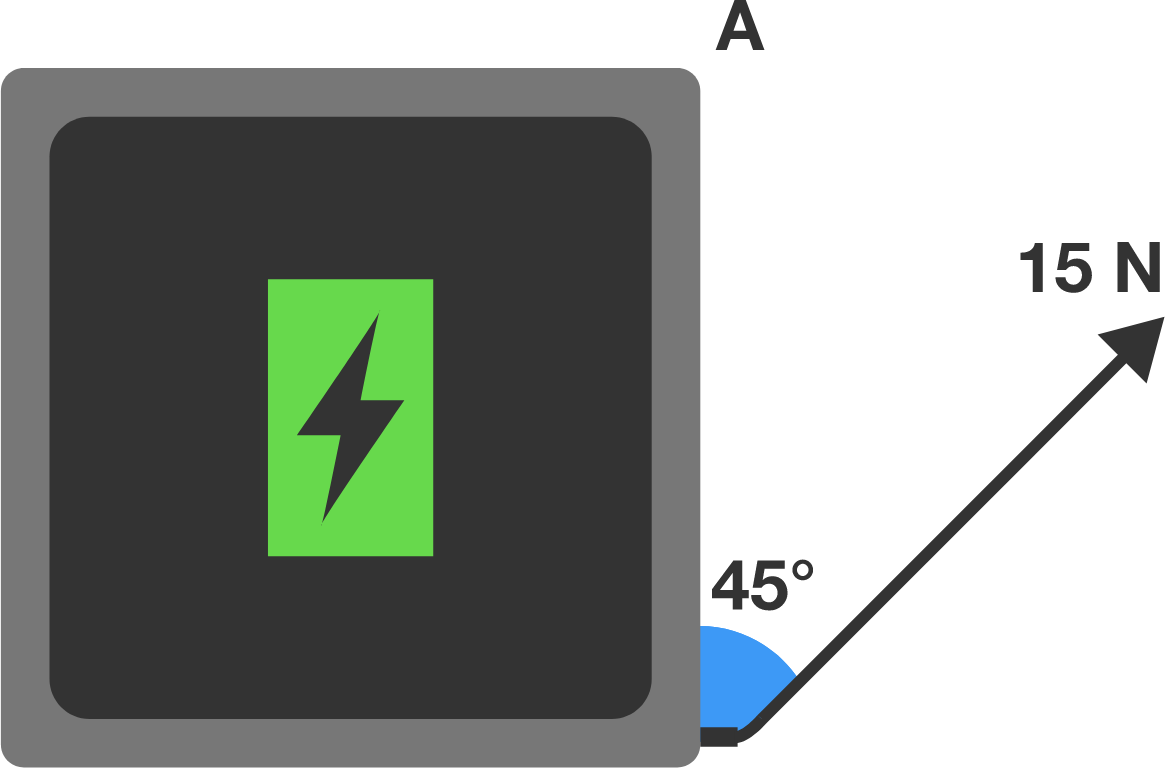# Problems of the Week

Contribute a problem

# 2018-03-12 Intermediate

Fraser, Georgina, and Owen are each trying to build the tallest snowman.

• Fraser: "Fewer snow spheres should be taller. I will stack 2 spheres of equal radii."
• Georgina: "I disagree, I think more snow spheres will be taller. I will stack 10 spheres of equal radii."
• Owen: "It doesn't matter how many spheres you make. I'll make 6 spheres of equal radii and all three of our snowmen will be the same height."

Who is correct?If they all have the same volume of snow, which snowman is tallest?

Details and Assumptions:

• Each child has the same volume of snow.
• Each sphere is perfectly spherical, and is tangential to the spheres above and below it. The bottom spheres sit on level ground.
• Assume that each child can stack the spheres so that they will not topple or collapse under the weight of the spheres above.

A fractal pattern is created in a square, beginning with 9 identical squares arranged in a plus sign pattern. At each step, every blue square that borders blue on exactly one edge is replaced with the plus sign pattern from the first step.As this pattern continues indefinitely, what fraction of the larger square is shaded blue?\begin{aligned} A &= \text{lcm} (1, \, 2, \, 3, \, \dots, \, 2018) \\\\ B &= \text{lcm} (1010, \, 1011, \, 1012, \, \dots, \, 2018) \end{aligned}

Which is true?


Notation: $\text{lcm}(\cdot)$ denotes the lowest common multiple function.Top view

A $1 \text{ kg}$ tablet with dimensions $30 \text{ cm} \times 30 \text{ cm}$ sits charging on a smooth table. The charging cord is attached to one of its corners. Somebody trips on the cord, applying a force of $15 \text{ N}$ to the tablet in the direction shown.

What is the initial acceleration at point $A$ in $\text{m/s}^2?$

Assume that tablet has uniform mass.

$1,\ 4,\ 9,\ 16,\ 25,\ 36,\ 49,\ 64, \ldots$

The above shows the first few perfect squares.

Except for 1 and 9, are there any other perfect squares whose digits are all odd numbers?

×